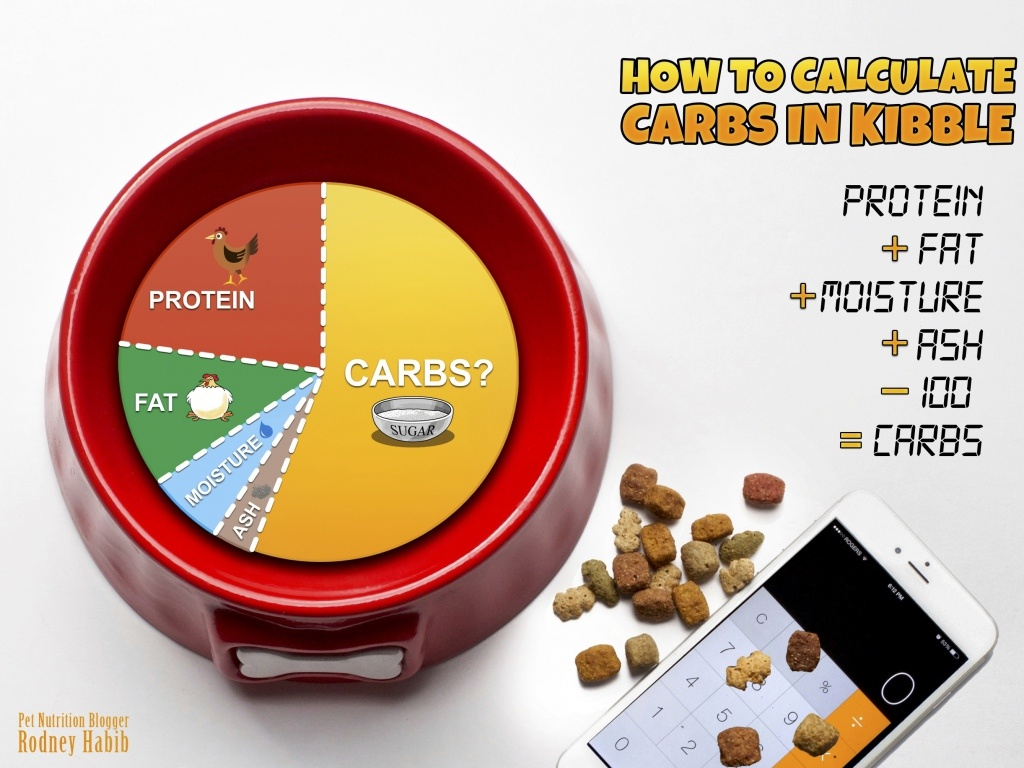A curated selection of elevated animal essentials for the modern pet parent. Orders \$75+ ship free.

# How to Calculate the Carb Content in High-Moisture (canned/wet) food

• Posted on
• By The Forever Dog by Rodney Habib & Dr. Karen BeckerMost pet parents don’t because the pet food manufacturer doesn’t really want you to know. Therefore, they don’t list it on the label. A very important figure to know, seeing the pet obesity rate is soaring over 60% and cancer (needs glucose to thrive), is roughly 1 in 2 pets.

First check this out:

According to Association of American Feed Control Officials (AAFCO): “Carbohydrates are not measured directly, but can be estimated by calculating the “nitrogen-free extract” in the product. This is determined simply by subtracting the average of each of the other components (percent crude protein, crude fat, crude fiber, moisture AND ash) from 100.” (If the ash is hidden, the general rule of thumb is between 5 to 8%, so pick a value.)

However experts say: “… fiber is actually a carbohydrate. So, its percentage is automatically included in your carbohydrate calculations.”

So let’s go with this guideline:

Protein + Fat + Moisture + Ash, then subtract 100 = Carbohydrates.

(This method works for dry food only; to figure out canned food you need to get the dry matter values - see below!)

After the calculations, pet parents are going to find that some of these bags of kibble have anywhere from 40% to 70% carbs in them. God forbid, if their pet has cancer (depending on the type of “cancer cell”) it can have anywhere from 10 to 70 times more insulin receptors on its cell surface membrane than a regular cell. Imagine: if cancer needs glucose for fuel and one is feeding their cancer pet that many carbohydrates, then in theory you are adding rocket fuel to the cancer growing fire!

Empower yourself and take better control of your pet’s diet!

#### How to Calculate the Carb Content in High-Moisture (canned/wet) food

To properly compare the guaranteed analysis of one food against another, you have to remove the water percentage from the equation and compare just the protein, fat, fiber, ash, and carb content This is called converting to a Dry Matter Basis (DMB).

#### The guaranteed analysis for our example canned food is:

• Protein 13%
• Fat 4%
• Fiber 2%
• Ash 1%
• Moisture 78%

STEP 1 - Subtract the moisture percentage (78%) from 100% to get 22% and convert that
to a decimal (24% = 0.24)

STEP 2 - Divide each of the other guaranteed analysis percentages by that decimal:

Protein = 13 / 0.22 = 59.09% DMB
Fat = 4 / 0.22 = 18.18% DMB
Fiber = 2 / 0.22 = 9.09% DMB
Ash = 1 / 0.22 = 4.55% DMB

To determine the carb content, subtract all of the DMB numbers above from 100. In this example, the DMB carb content is 9.09%.

#### How to Calculate the Carb Content in Dry Foods

To determine the carb content in dry foods, add up the protein, fat, fiber, moisture and ash from the guaranteed analysis and subtract from 100. If ash is not listed, use 6% as a general average for dry food. This equals the carb/starch content.

#### The guaranteed analysis for our example canned food is:

• Protein 26%
• Fat 14%
• Fiber 4%
• Ash 6%
• Moisture 10%

Add: 26% + 14% + 4% + 6% + 10% = 60%

Subtract from 100: 100% – 60% = 40%

The carb content of our sample dry food is 40%